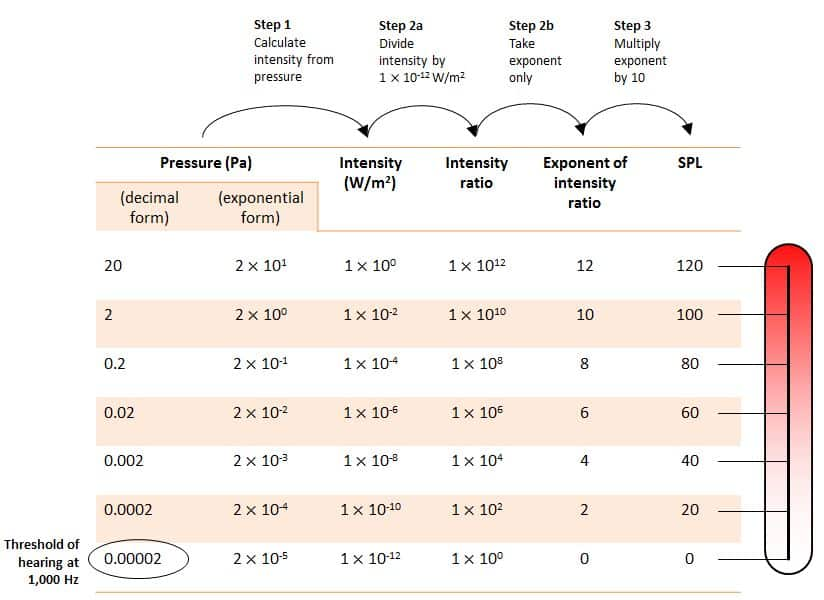»» Lesson 2: Sound pressure level (SPL): Our way to quantify strength of noise (201912A)

## Lesson 2: Sound pressure level (SPL): Our way to quantify strength of noise (201912A)

Lesson 2

Sound Pressure Level (SPL): Our Way to Quantify The Strength of Noise

After we have comprehended what background noise is, let’s talk about how we quantify it.

It’s fairly commonplace for us to describe the loudness of sound, including noise, with qualitative terms, such as loud or quiet. But, can you imagine how confused you would be if your friend said that his washing machine generates a ‘loud’ squeaky noise when spinning? You would wonder how loud it is. It’s okay that he couldn’t describe it with a number, but the problem here lies in the fact that he neglected the reference.

Since we need to measure it (objectively), scientists and engineers introduce a more ‘responsible’ term, and that is sound pressure level. However, keep in mind, this quantity alone doesn’t represent our psychoacoustic perception of loudness. It’s more about the physical strength of sound pressure. This is what’s in the display of measuring instruments.

Figure 1 Measuring noise using SPL-meter

# Sound pressure level (SPL): The definition

Let’s recall the meaning of pressure a bit. We know from school that the definition of pressure is the force applied divided by the area over which that force is perpendicularly distributed.Figure 2 Pressure = Force / Area

In the International System of Units (SI), a force is expressed in newton (N) while an area is in a square meter (m2). Therefore, the SI unit of pressure is N/m2, and it’s called pascal (Pa), named after a French physicist, Blaise Pascal.

The threshold of hearing for a human ear at 1,000 Hertz (Hz) corresponds to the sound pressure of 0,00002 Pa. This number was set by scientists after some scientific research that incorporates thousands of measurements among people from various age, gender, and racial groups. Meanwhile, the highest sound pressure before human ears feel pain is 20 Pa.

# Why and how did scientists change pascal to decibel?

Most people will have problems if they have to express the magnitude of sound pressure from 0,00002 Pa to 20 Pa. Therefore, some scientists sat together to find a new quantity to express the strength of sound pressure in a more simple number. After several numbers of math games, they conclude the solution as depicted below.Figure 3 Sound pressure level conversion

## The first step, they converted the sound pressure (Pa) to the sound intensity (W/m2).

When traveling in the air, sound with the pressure of 20 Pa is equivalent to that with 1 W/m2 of sound intensity, as shown in Figure 3 above. As sound intensity is proportional to the square of its pressure, we can derive the sound intensity at the other pressure. We can see here that the exponents of sound intensity for 20 Pa and 0.00002 Pa are 0 and -12, respectively. However, they didn’t want to have a negative value. Hence, they made the intensity at the threshold of hearing as the reference value. It then brings us to the second step.

## The second step, they divided every sound intensity by this reference value so that we can get the intensity ratio.

From here, each intensity ratio value appears to be more simple than any other numbers. We have the exponents that are all positive. Now, let’s take the exponents only and guess what we have! We have a range of exponent values from 0 to 12. However, this is still too narrow if we want to correlate this number with the human perception of loudness. That brings us to the next step.

## The third step, scientists multiplied each exponent value by 10 (deci in Latin) to make this number more relevant to human perception.

Now, the range is from 0 to 120. If the number is increased by 3, people start to notice the difference. They eventually decided to stick with this range. Next, they call this new quantity as the sound pressure level.

Like any other quantities, we need a unit to represent this value. After brainstorming for awhile looking for the best name, they agreed to use bel (from Alexander Graham Bell, the inventor of the first practical telephone) to honour him. So, now we have decibel (dB) as the unit for this logarithmic scale to represent the sound pressure level.

# Sound pressure level and human perception of loudness

In acoustics, sound pressure level and loudness are two distinct quantities, but they indeed have a strong correlation. One of the factors that influences the strength of loudness is the sound pressure level. The loudness is more about how our human ears perceive the strength of sound pressure. This is what makes it interesting.

Let’s see Figure 4 below. If 60 dB of noise had the same loudness across all frequencies, the loudness curve would follow the straight line as depicted by the green line. In reality, loudness doesn’t work that way. The amount of sound pressure level at 1,000 Hz will have different loudness with that at 20 Hz. The approximation of loudness in various frequencies is shown by the purple contours. These contours are often called as the equal-loudness contours, the more developed version of the Fletcher–Munson curves.

Figure 4 ISO 226 equal-loudness contours (Mathworks with modification)

Let’s take an example. See the contour highlighted in red. 60 dB of noise at 1,000 Hz will have the same loudness as 78 dB and 55 dB of noise at 100 Hz and 3,000 Hz respectively.

Now, you have learned two important things today.
1. We can use the sound pressure level to quantify any sound, including noise.
2. The same sound pressure level can have different loudness at different frequencies.